• +91 9971497814
• info@interviewmaterial.com

# RD Chapter 22- Brief Review of Cartesian System of Rectangular Coordinates Ex-22.1 Interview Questions Answers

### Related Subjects

Question 1 :

If the line segmentjoining the points P(x1,y1) and Q(x2, y2) subtends an angle α at the origin O, prove that: OP. OQ cos α = x1 x2 + y1 y2.

Given,

Two points P and Q subtends an angle α at the origin as shown infigure: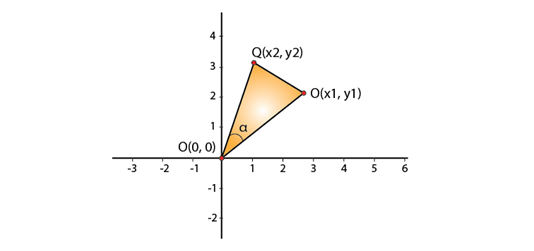From figure we can see that points O, P and Q forms a triangle.

Clearly in ΔOPQ we have: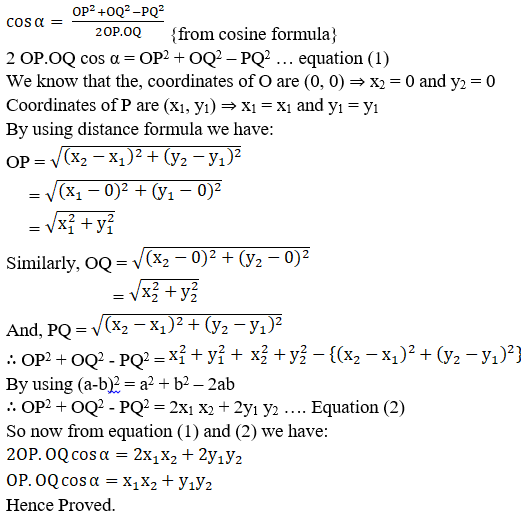Question 2 : The vertices of a triangle ABC are A(0, 0), B (2,-1) and C (9, 0). Find cos B.

Given:

The coordinates of triangle.

From the figure,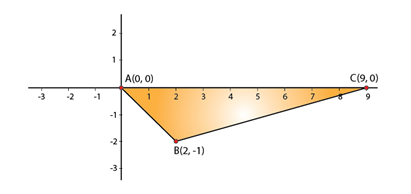By using cosine formula,

In ΔABC, we have: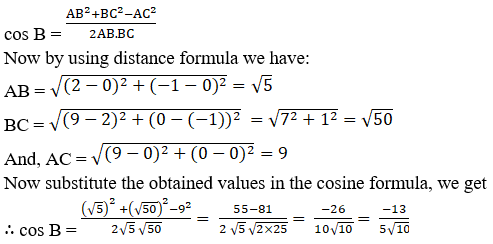Question 3 :

Four points A (6,3), B (-3, 5), C (4, -2) and D (x, 3x) are given in such a way that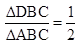, find x.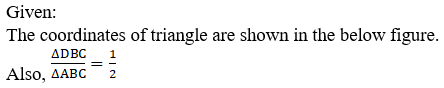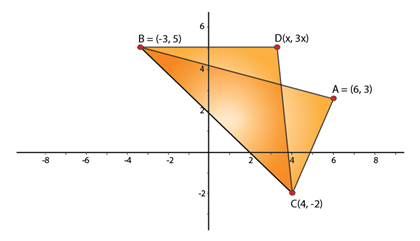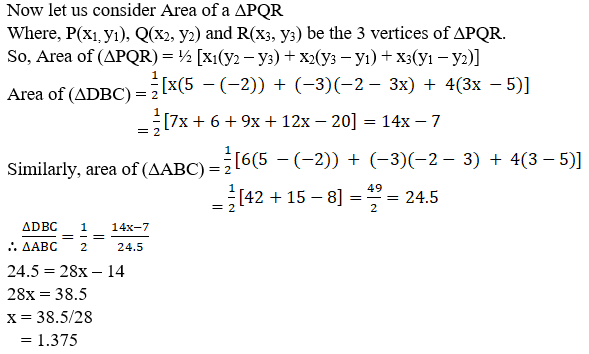24.5 = 28x – 14

28x = 38.5

x = 38.5/28

= 1.375

Question 4 :

The points A (2,0), B (9, 1), C (11, 6) and D (4, 4) are the vertices of a quadrilateral ABCD.Determine whether ABCD is a rhombus or not.

Given:

The coordinates of 4 points that form a quadrilateral is shownin the below figure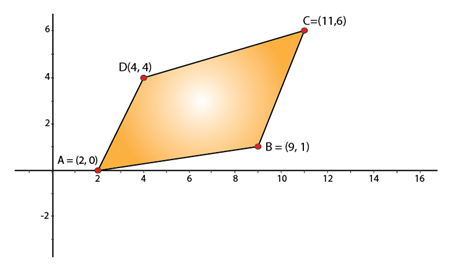Now by using distance formula, we have: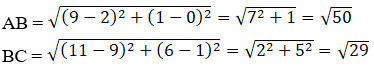It is clear that, AB ≠ BC [quad ABCD does not have all 4sides equal.]

ABCDis not a Rhombus

Todays Deals### RD Chapter 22- Brief Review of Cartesian System of Rectangular Coordinates Ex-22.1 Contributorskrishan

Name:
Email:

# Latest News# 9000 interview questions in different categories